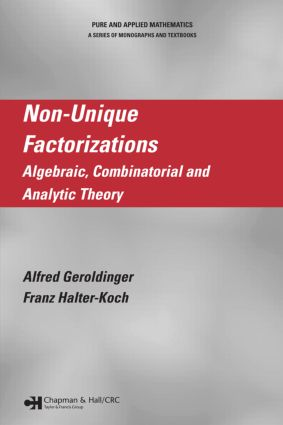# Non-Unique Factorizations

## Algebraic, Combinatorial and Analytic Theory, 1st Edition

Chapman and Hall/CRC

728 pages | 7 B/W Illus.

##### Purchasing Options:\$ = USD
Hardback: 9781584885764
pub: 2006-01-13
SAVE ~\$37.00
\$185.00
\$148.00
x
eBook (VitalSource) : 9780429139567
pub: 2006-01-13
from \$28.98

FREE Standard Shipping!

### Description

From its origins in algebraic number theory, the theory of non-unique factorizations has emerged as an independent branch of algebra and number theory. Focused efforts over the past few decades have wrought a great number and variety of results. However, these remain dispersed throughout the vast literature. For the first time, Non-Unique Factorizations: Algebraic, Combinatorial, and Analytic Theory offers a look at the present state of the theory in a single, unified resource.

Taking a broad look at the algebraic, combinatorial, and analytic fundamentals, this book derives factorization results and applies them in concrete arithmetical situations using appropriate transfer principles. It begins with a basic introduction that can be understood with knowledge of standard basic algebra. The authors then move to the algebraic theory of monoids, arithmetic theory of monoids, the structure of sets of lengths, additive group theory, arithmetical invariants, and the arithmetic of Krull monoids. They also provide a self-contained introduction to abstract analytic number theory as well as a modern treatment of W. Narkiewicz's analytic theory of non-unique factorizations.

Non-Unique Factorizations: Algebraic, Combinatorial, and Analytic Theory builds the discussion from first principles to applied problem solving, making it ideally suited to those not familiar with the theory as well as those who wish to deepen their understanding.

### Reviews

"Combining methods of various branches of mathematics, it brings together a theory from classical results to topics reflecting the recent ideas. It is a nice book written in a precise, readable style."

– In EMS Newsletter, September 2007

CONCEPTS IN FACTORIZATION THEORY AND EXAMPLES

Atoms and Primes

Free Monoids, Factorial Monoids and Factorizations

BF-Monoids

Systems of Sets of Lengths

FF-Monoids

The Catenary Degree and the Tame Degree

Rings of Integers of Algebraic Number Fields

ALGEBRAIC THEORY OF MONOIDS

v-Ideals

Prime Ideals and Localizations

Complete Integral Closures and Krull Monoids

Divisor Homomorphisms and Divisor Theories

Krull Monoids and Class Groups

Defining Systems and v-Noetherian Monoids

Finitary Monoids

Class Semigroups

C-Monoids and Finitely Primary Monoids

Integral Domains

Congruence Monoids and Orders

ARITHMETIC THEORY OF MONOIDS

Finitary Monoids

Transfer Principles

C-Monoids

Saturated Submonoids and Krull Monoids

Type Monoids

Faithfully Saturated Submonoids

Integral Domains and Congruence Monoids

Factorizations of Powers of an Element

THE STRUCTURE OF SETS OF LENGTHS

Multidimensional Arithmetical Progressions

Almost Arithmetical Multiprogressions

An Abstract Structure Theorem for Sets of Lengths

Pattern Ideals and Complete s-Ideals in Finitary Monoids

Products of Strongly Primary Monoids and their Submonoids

C-Monoids

Integral Domains and Congruence Monoids

Realization Theorems and Further Examples

Sets of Lengths of Powers of an Element

Sequences over Abelian Groups

Zero-Sumfree Sequences

Cyclic Groups

Group Algebras and p-Groups

Coverings by Cosets and Elementary p-Groups

Short Zero-Sum Sequences and the Inductive Method

Groups of Rank Two

ARITHMETICAL INVARIANTS OF KRULL MONOIDS

The Generalized Davenport Constants

The Narkiewicz Constants

The Elasticity and Its Refinements

The Catenary Degree

The Tame Degree

Sets of Lengths Containing 2

The Set of Distances and Maximal Half-Factorial Sets

Minimal Non-Half-Factorial Sets

GLOBAL ARITHMETIC OF KRULL MONOIDS

Arithmetical Characterizations of Class Groups I

Arithmetical Characterizations of Class Groups II

The System of Sets of Lengths for Finite Abelian Groups

The System of Sets of Lengths for Infinite Abelian Groups

Additively Closed Sequences and Restricted Sumsets

Factorization of Large Elements

ABSTRACT ANALYTIC NUMBER THEORY

Dirichlet Series

A General Tauberian Theorem

Abstract Formations and Zeta Functions

Arithmetical Formations I: Zeta Functions

Arithmetical Formations II: Asymptotic Results

Arithmetical Formations III: Structure Theory

Geometrical Formations I: Asymptotic Results

Geometrical Formations II: Structure Theory

Algebraic Function Fields

Obstructed Formations

ANALYTIC THEORY OF NON-UNIQUE FACTORIZATIONS

Analytic Theory of Types

Elements with Prescribed Factorization Properties

The Number of Distinct Factorizations

Block-Dependent Factorization Properties

APPENDIX A: ABELIAN GROUPS

APPENDIX B: COMPLEX ANALYSIS

APPENDIX C: THEORY OF INTEGRATION

APPENDIX D: POLYHEDRAL CONES

BIBLIOGRAPHY

LIST OF SYMBOLS

SUBJECT INDEX

### Subject Categories

##### BISAC Subject Codes/Headings:
MAT002000
MATHEMATICS / Algebra / General
MAT003000
MATHEMATICS / Applied
MAT036000
MATHEMATICS / Combinatorics